# Compound Interest - Quantitative Aptitude (MCQ) questions

Dear Readers, Welcome to Quantitative Aptitude Compound Interest questions and answers with explanation. These Compound Interest solved examples with shortcuts and tricks will help you learn and practice for your Placement Test and competitive exams like Bank PO, IBPS PO, SBI PO, RRB PO, RBI Assistant, LIC,SSC, MBA - MAT, XAT, CAT, NMAT, UPSC, NET etc.

After practicing these tricky Compound Interest multiple choice questions, you will be exam ready to deal with any objective type questions.

1)   What will be ratio of simple to compound interest on two same sums invested in SBI at rate of interest of 8% kept for 3 years?
- Published on 08 May 17

a. 1875/2029
b. 1/2.5
c. 1903/2156
d. 4/9
 Answer  Explanation ANSWER: 1875/2029 Explanation: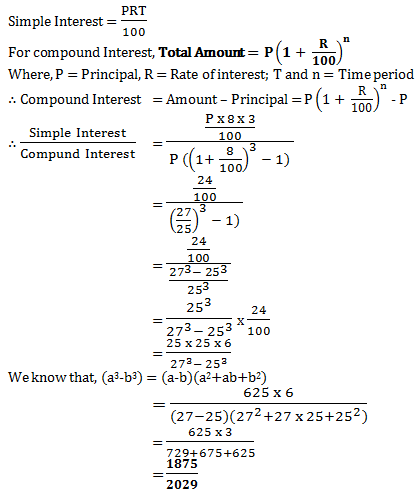2)   Raju invested Rs. 77500 in ICICI bank. In two years how much compound interest will he get, if the first year rate of interest was 10% and second year had 2% more than first year?
- Published on 08 May 17

a. Rs. 17850
b. Rs. 17980
c. Rs. 18963
d. Rs. 16880
 Answer  Explanation ANSWER: Rs. 17980 Explanation: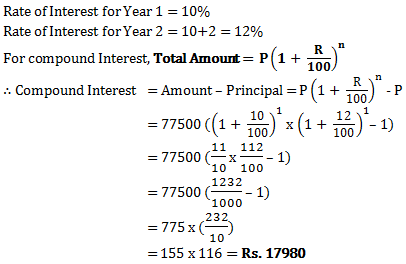3)   What will be difference in population 3 years ago and 2 years ago of Devon village, whose current population is 100000 and which is increasing at a rate of 25% every year?
- Published on 08 May 17

a. 15250
b. 13900
c. 16400
d. 12800
 Answer  Explanation ANSWER: 12800 Explanation: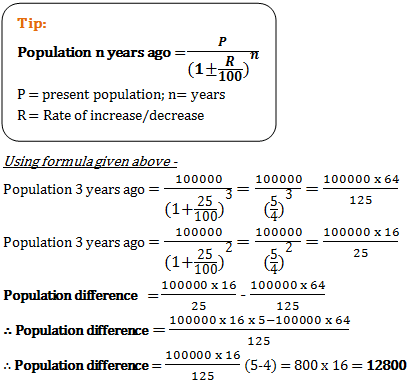4)   A has two grandsons P and Q. 13 year old P gets some money from A’s wealth and 14 year old Q gets rest of the money. But P and Q will get money only when they turn 25 years old. Till then the money is in a bank getting interest at rate 4% compounded annually. When both turn 25, they receive the same amount. How much had A given Q initially, if total money with A was Rs.25500?
- Published on 08 May 17

a. Rs. 12500
b. Rs. 13000
c. Rs. 15000
d. Rs. 11500
 Answer  Explanation ANSWER: Rs. 13000 Explanation: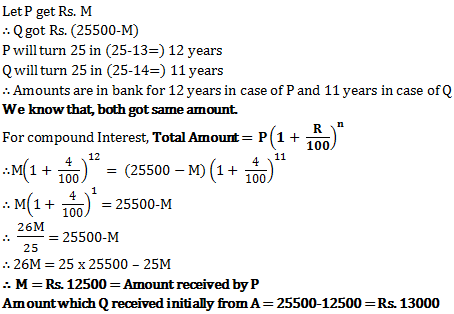5)   Raj has Rs. 1301 with him. He divided it amongst his sons Prakash and Prashant and asked them to invest it at 4% rate of interest compounded annually. It was seen that Prakash and Prashant got same amount after 17 and 19 years respectively. How much did Raj give to Prashant?
- Published on 03 May 17

a. Rs. 705
b. Rs. 615
c. Rs. 676
d. Rs. 625
 Answer  Explanation ANSWER: Rs. 625 Explanation: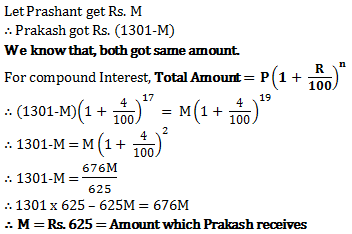6)   Shamik had invested same amount of sums at simple as well as compound interest. The time period of both the sums was 2 years and rate of interest too was same 4% per annum. At the end, he found a difference of Rs. 50 in both the interests received. What were the sums invested?
- Published on 03 May 17

a. Rs. 32550
b. Rs. 35750
c. Rs. 30000
d. Rs. 31250
 Answer  Explanation ANSWER: Rs. 31250 Explanation: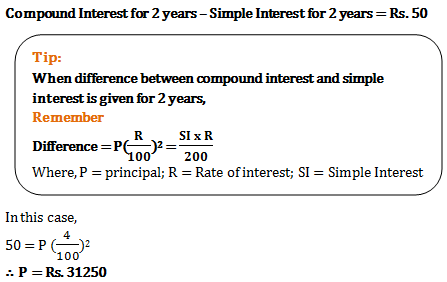7)   Sarang invested some money in HDFC at 3% rate of interest. What would be the corresponding simple interest if after 2 years, Sarang got Rs. 101.50 as compound interest?
- Published on 03 May 17

a. Rs. 1015
b. Rs. 125
c. Rs. 100
d. Rs. 150
 Answer  Explanation ANSWER: Rs. 100 Explanation: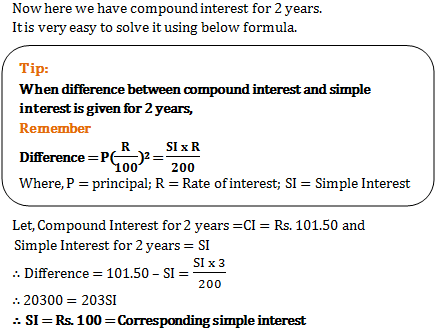8)   Ramesh gets double the amount in 9 years when invested at compound interest. In how many years will the amount become four times itself?
- Published on 13 Apr 17

a. 13.5 years
b. 27 years
c. 9 years
d. 18 years
 Answer  Explanation ANSWER: 18 years Explanation: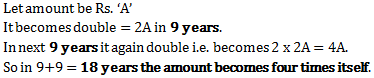9)   When difference between compound and simple interest for three years is Rs. 122 at 5% rate per annum, the principal is ______
- Published on 12 Apr 17

a. Rs. 24400
b. Rs. 14400
c. Rs. 18000
d. Rs. 16000
 Answer  Explanation ANSWER: Rs. 16000 Explanation: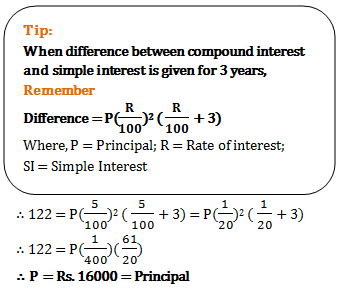10)   If at same rate of interest, in 2 years, the simple interest is Rs. 40 and compound interest is Rs. 41, then what is the principal?
- Published on 12 Apr 17

a. Rs. 500
b. Rs. 400
c. Rs. 800
d. Rs. 820
 Answer  Explanation ANSWER: Rs. 400 Explanation: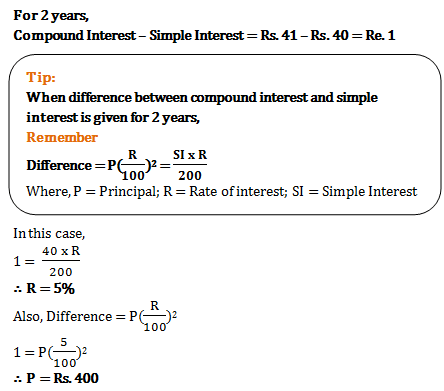1 2 3